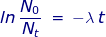# Rate of Decay Formula

Rate of Decay Formula

The rate of decay for radioactive particles is a first order decay process. This means it follows an exponential decay pattern which can be easily calculated.Nt = the amount of radioactive particles are time (t)

N0 = the amount of radioactive particles at time = 0

λ = rate of decay constant

t = time

Taking the natural log of both sides of this equation (remember it is an exponential decay) will yield:Rate of Decay Formula Questions:

1. Calculate the rate of decay constant for U-238 if its half-life is 4.468 × 109 years.

If the problem is referring to the half-life, then the ratio of= 0.5 because half of the original sample has already undergone decay.1.55 x 10-10 years-1

2. Calculate how long it will take for 25% of a U-238 sample to radioactively decay.

If 25% of the sample has undergone radioactive decay, then the ratio of= 0.75 because 75 percent of the original sample remains. The rate of decay constant for U-238 will be used from the previous problem.1.86 x 109 years = t